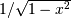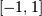# numpy.polynomial.chebyshev.chebweight¶

numpy.polynomial.chebyshev.chebweight(x)[source]

The weight function of the Chebyshev polynomials.

The weight function isand the interval of integration is. The Chebyshev polynomials are orthogonal, but not normalized, with respect to this weight function.

Parameters: x : array_like Values at which the weight function will be computed. w : ndarray The weight function at x.

Notes

New in version 1.7.0.

#### Previous topic

numpy.polynomial.chebyshev.chebgauss

#### Next topic

numpy.polynomial.chebyshev.chebcompanion